# Dereference Pointers in C++

Muhammad Husnain Feb 03, 2023 Apr 20, 2022

This tutorial is a brief discussion on dereferencing pointers in C++.

Before moving to our actual topic, we first need to understand what a pointer is and why C++ programmers come across the need for it.

## Pointers in C++

In C++, variables are considered the memory locations accessed by their identifiers. By this method, a programmer is unaware of the physical address of the memory where that particular variable is stored and accesses it by its identifier or variable name.

In C++ programs, physical memory is a series of memory cells with each cell of one-byte size. When a variable with more than one byte is saved in memory, it takes consecutive memory locations.

For instance, an `int` variable is four bytes in size, so it takes four consecutive memory cells to store an integer value.

When a variable is declared in C++, its required amount of memory is assigned to a particular address (known as its physical address). This is generally decided on run-time by the operating system or the program’s environment where that physical address is.

This memory address can be accessed and stored in some variable. We use an operator known as the `address-of` operator to access it, denoted by the symbol `&`.

## Use the `&` Operator to Obtain Memory Address in C++

The `address-of` operator obtains the memory address where a particular variable is stored. It is used by preceding the variable name with the `&` operator.

See the code snippet below:

``````addr = &newvar
``````

By this, the address of the variable `newvar` will be assigned to the variable `addr` but not the content. This is depicted in the figure below.

Example:

``````newvar = 25;
``````

Output: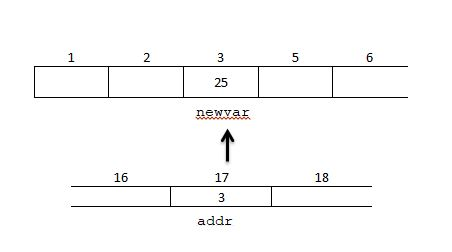In this code, first, we assigned the value `25` to the variable `newvar`, and `newvar` is stored at the memory location `3`. Then we have assigned the address of `newvar` to the variable `addr`.

So, the `addr` variable in which we stored the address of `newvar` has the value `3`, the memory address of `newvar`.

## Declare Pointers in C++

The variable used to store the address of another variable is referred to as a pointer variable. In the example above, the `addr` variable is a pointer.

Pointers are very powerful features in programming. A pointer variable of a particular type points to a variable of the same type.

It is declared using an asterisk (`*`) operator at the time of declaration of the pointer variable. Consider the code snippet below:

``````#include<iostream>
using namespace std;

int main(){
int num = 5;
int * pNum;

pNum = &num;
cout<<"Value of num is: "<<num<<endl;
return 0;

}
``````

Output: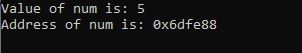The program output shows the value of `newvar` that is `5` and its address stored in the `pNum` pointer.

Because of the ability of pointers to directly point to the variable value, they need to have a data type that is the same as the variable to which they point.

Syntax:

``````datatype * nameOfPointer;
``````

Here, the `data type` is the type of the variable to which it points.

Example:

``````int * numPtr;
char * charPtr;
double * doublePtr;
``````

There are three declarations of pointers in this code snippet, but they are of different types. They are intended to occupy the same amount of space in the memory (the amount of space depends on the program’s environment).

Neither of them can point to a different type of variable.

## Use the `*` Operator to Dereference Pointer in C++

In the previous example, we have used the `address-of` operator to store the address of the variable in the pointer. The dereference operator (`*`) gets the contents of a variable to which the pointer is pointing.

We can get the variable value whose address is saved in the pointer.

In the example below, we access the variable `newvar` value by dereferencing the pointer and directly using the variable. We can observe in the output that both are the same.

``````#include<iostream>
using namespace std;

int main(){
int num = 5;
int * pNum;

pNum = &num;
cout<<"Value of num is: "<<num<<endl;
cout<<"Value of num by using pointer: "<<*pNum<<endl;
return 0;
}
``````

Output: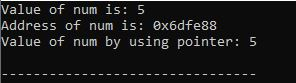We can also save the value that is dereferenced in some other variable like this:

``````num2 = *pNum;
``````

This means that `num2` equals the variable value pointed by `pNum`. This is demonstrated in the following figure.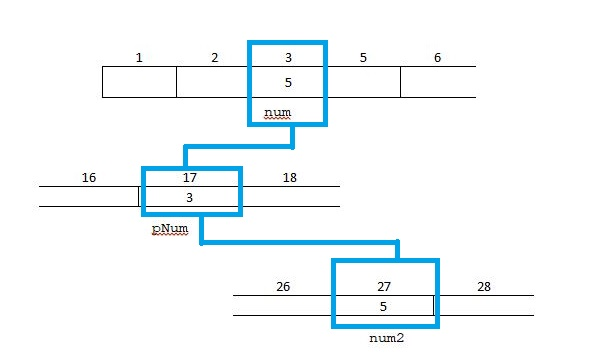Here, `num2` is assigned the same value as `num` using the dereference operator.

### Does Dereferencing Make a Copy

Dereferencing sometimes makes a copy if we do more than dereferencing. Hence, if we use that dereferenced value to initialize a new variable, that is a copy, as shown in the above example.

But if we use the dereferenced value and save it as a reference in some reference variable, then that is not a copy, but an alias is created.

``````int num = 5;
int *pNum = &num;
int& num2 = *pNum;
``````

The dereference operator initialized the reference variable `num2` in the last line. This reference variable `num2` will now contain the address of `num` and is an alias of `num`. It does not copy its value.

This is explained in the figure below.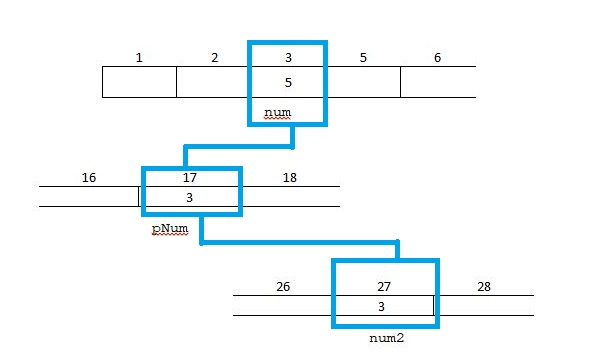Now, `num2` also points to `num` because it contains the memory address of `num`. Thus, pointers are a helpful tool in programming and provide us with efficient memory and time management during programming.

Husnain is a professional Software Engineer and a researcher who loves to learn, build, write, and teach. Having worked various jobs in the IT industry, he especially enjoys finding ways to express complex ideas in simple ways through his content. In his free time, Husnain unwinds by thinking about tech fiction to solve problems around him.